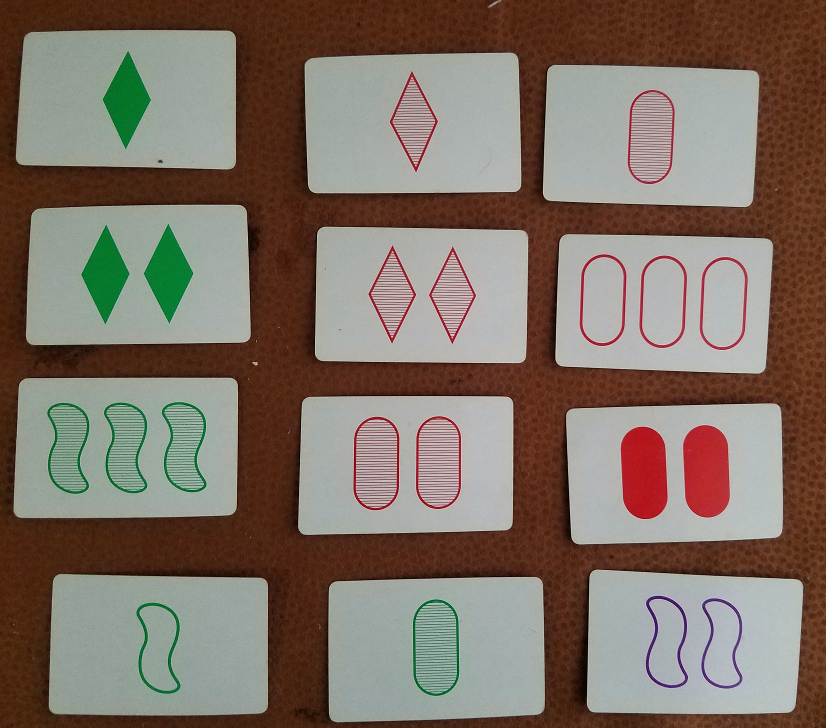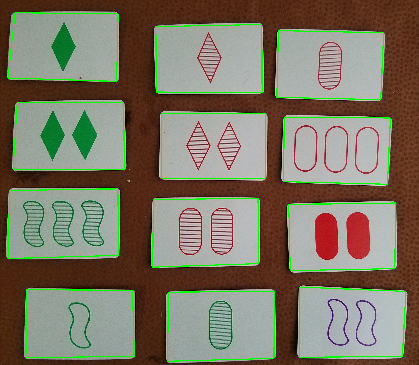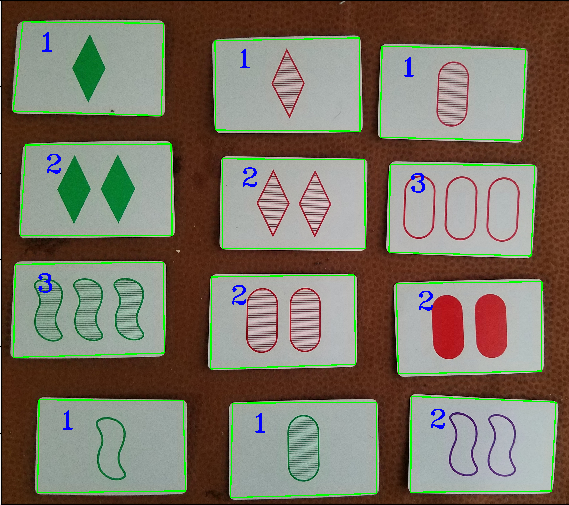# Solving the Game of SET: Part I

### Game of SET

SET is a visual perception game consisting of a deck of 81 cards each with a combination of 4 features - shape (squiggle, diamond, or oval), fill (solid, empty, or hash), number (one, two or three), and color (red, green, or purple). 3^4 = 81 different cards. The game is played by shuffling the deck and dealing 12 cards out in a grid. A SET is made by combining three cards that have either share a common feature or are all different for that feature. For example, in the game below the red ovals in the upper right (single hash red oval, three empty red ovals, and two solid red ovals) form a set because they all either share a common feature (color, shape), or have all different features (fill, number).

This game is really hard for me. No matter my attempt at a strategy, I always end up panicking and scanning frantically. Combine my difficulty with my wife’s incredible speed and you got a computer vision project brewing!

### Project Overview

The goal of this project is to build a SET solving program using Python, OpenCV, and a webcam to generate solutions to displayed SET boards. Some constraints:

• Analysis should happen in realtime - no taking pictures and uploading for analysis.
• Setup should be robust to various lighting conditions and viewing angles

Because the cards are pretty simple, I decided to use deterministic methods for card identification and analysis. Each card grid (which I’m calling a scene) will be processed to:

• Find and count the number of cards present
• Segment each card for individual processing of:
• Symbol Count
• Symbol Shape
• Symbol Color
• Symbol Fill
• Using these values, game solutions will be solved and displayed.

The program will be seperated into three major components:

• ProcessScene
• Find each of our cards
• ProcessCards
• ID the features of the card
• PlayGame
• Solve the game and return the solution

#### Identifying Cards - ProcessScene

The first step here is to find the number of cards in the scene. HSV filtering works really well here, especially when the glare coming off the glossy cards is minimized and the game is played on a non-white background. Here’s the result of HSV filtering on an example game:Example game and the corresponding HSV filtered image.

We can easily use this image to run some contours and extract our image. After extracting the contours, I only keep the contours that have four points and exceed a pre-set threshold. I also check to make sure that the shape we’ve found is a rectangle. This is done by calculating the four corner angles. With a skewed viewing angle, they won’t necessarily be perfect right angles, but they should fall in a range.

def is_rect(pts):
pts = np.array(pts).reshape(4, 2)
pts = order_pts(pts)
x_pts = np.append(pts[:, 0], pts[0, 0])
y_pts = np.append(pts[:, 1], pts[0, 1])

# Calculate all four angles
theta = np.arctan2(np.diff(x_pts), np.diff(y_pts))

# Correct angles based on initial line segment
theta = abs(angle_trunc(theta - theta))
# print (theta)
angle_min = 0.7 * pi / 2
angle_max = 1.3 * pi / 2

angle_test = np.where(np.logical_or(np.logical_and(theta >= 0, theta < 0.1),
np.logical_and(theta > angle_min, theta < angle_max)))

if len(angle_test) == 4:
return True

else:
return False


Combined with our HSV filter and Gaussian blurring, this eliminates a lot of noise and false positives from our search space. Once our cards are extracted, we warp them to a fixed size to correct for camera angles. This also makes our life a lot easier when extracting shapes later as the shapes will all be the same size and un-skewed. We have to do some trickier to account for images that could be in view but sideways, we don’t want to accidently force a rotated card to fit in the box we’ve defined.

def warp_cards(c, img):
new_width = 100
new_height = (2 / 3.) * new_width
pts = c.reshape(4, 2)
rect = np.zeros((4, 2), dtype="float32")
s = pts.sum(axis=1)
rect = pts[np.argmin(s)]
rect = pts[np.argmax(s)]

diff = np.diff(pts, axis=1)
rect = pts[np.argmin(diff)]
rect = pts[np.argmax(diff)]
(tl, tr, br, bl) = rect

top_len = np.linalg.norm(tl-tr)
side_len = np.linalg.norm(tr-br)

if side_len > top_len:
rect = np.roll(rect, 1, axis=0)
(tl, tr, br, bl) = rect

maxWidth = int(new_width)
maxHeight = int(new_height)
# construct our destination points
dst = np.array([
[0, 0],
[maxWidth - 1, 0],
[maxWidth - 1, maxHeight - 1],
[0, maxHeight - 1]], dtype="float32")
# warp the image
M = cv2.getPerspectiveTransform(rect, dst)
warped = cv2.warpPerspective(img, M, (maxWidth, maxHeight))
return warped


Now all our cards are isolated and each card has been extracted, warped to the same size and rotated to the same angle. The order is different from that displayed in the scene, but this isn’t actually important as the game doesn’t depend on the location of the cards relative to each other. All we need to do is keep the location consistent between frames - this is important when we move to a webcam instead of still images - and maintain the (x,y) coordinates of the card in the scene.Bounding box around each card and the grid of cards found, isolated, and warped.

#### Identifying Card Features - ProcessCard

Once the cards have been isolated, we can being identifying the features of the card. Some of these features are pretty trivial to find and the methods produce very robust results (card count and symbol shape). The other features (card color and card fill) are a bit less robust and more difficult to identify correctly. Some of this is because of the quality of the webcam - hatch fill can get blurred by a low quality image.

##### Find Number of Symbols

This is done using contours to extract the symbol outlines and count the number of features extracted. Much like the cards, we use an area threshold to avoid getting small contours and then count the result. This one was pretty easy and very robust.

##### Find Shape of Symbols

Finding the shape was a bit trickier than the count. Diamonds are really straightforward because of their distinct points. We can easily look at the contours and count the number of points in line approximation. Squiggles and ovals are trickier to tell apart with this method. I ended up using a simple error metric between a template image and the found image.Scene with symbol count and shape identified.
def find_shape(self):
side_array = []
if self.contours is not None:
for pts in self.contours:
side_array.append(len(pts))
side_mean = np.mean(side_array)
self.bw_sym_img = bw_filter(self.symbol_img)
self.thresh_sym = threshold_img(self.bw_sym_img)
self.filled_sym = flood_fill(self.thresh_sym)
if 3.5 < side_mean < 6.0:
shape_str = 'Diamond'
shape_idx = 0
else:
err = []
for shapes in TEMPLATE_ARRAY:
cmp_shape = self.resize_to_shape(shapes, self.filled_sym)
err.append(ssim(cmp_shape, self.filled_sym))
err = np.array(err)
shape_idx = np.argmax(err) + 1
shape_str = TEMPLATE_ARRAY[np.argmax(err), 1]

self.shape = shape_idx
self.shape_str = shape_str

##### Find Color and Fill of Symbols

Color was really tricky. I tried a number of methods that achieved mediocre results, at best. HSV filtering wasn’t robust enough to identify the colors of non-solid shapes. The problem I was facing is that color is constrained to only small regions of the empty/hash cards. Because the empty card is almost entirely white, I first needed to find a way to extract just the colored region. I settled on using a bitwise mask to extract the colored parts using a thresholded version of the card. This does a pretty reasonable job of extracting just the colored region.

Using this image, I estimate the mean of the vibrance channel from the HSV image. I’ve created some bins for each color based on the hue value and add each pixel based on which color range it falls in. Whichever color bin has the highest count is chosen as the correct color.

def find_color(self):
sym_hsv = cv2.cvtColor(self.sym_mask, cv2.COLOR_BGR2HSV)
norm_hsv = cv2.cvtColor(self.normalize_patch, cv2.COLOR_BGR2HSV)
norm_mean = np.mean(norm_hsv[:, :, 0], axis=(0, 1))
hue = sym_hsv[:, :, 0]
sym_mean = hue[hue > 0].flatten()

purple_mean = ((sym_mean > 115) & (sym_mean < 160)).sum()
green_mean = ((sym_mean > 60) & (sym_mean < 90)).sum()
red_mean = ((sym_mean > 1) & (sym_mean < 10)).sum() + (sym_mean > 175).sum()
color_choice = np.array([red_mean, green_mean, purple_mean])

self.color = np.argmax(color_choice)
self.color_str = COLOR_STR[self.color]


I ended up being pretty pleased with these results and found really robust identification even with the empty and hash cards.

To find the fill of the image, I extract a small patch from the center of the symbol and a small patch from the corner to use as an example of a known color (white). Solid fills are pretty easy to determine based on the mse between the extracted patch and the normal patch. Hash and empty patches are tougher to distinguish between based on the poor webcam quality. I get good results from using a Canny edge detector and then checking if there are stripes in the extracted patch.

def find_fill(self):
width = 4
height = 4
h, w, d = self.symbol_img.shape

h_min, h_max = int(h/2) - height, int(h/2) + height
w_min, w_max = int(w/2) - width, int(w/2) + width

self.normalize_patch = self.warp[0:2 * height, 0:2 * width, :]
self.fill_patch = self.symbol_img[h_min:h_max, w_min:w_max]

normal_mean = np.mean(self.normalize_patch, axis=(0,1))
patch_mean = np.mean(self.fill_patch, axis=(0,1))
distance = mse(normal_mean, patch_mean)

sym_edge = cv2.Canny(self.fill_patch, 10, 50)
fill_cnt = (sym_edge > 0).sum()

if distance > 100:
self.fill = 2
self.fill_str = 'Solid'

elif 0 <= fill_cnt <= 5:
self.fill = 0
self.fill_str = 'Empty'

else:
self.fill = 1
self.fill_str = 'Hash'


#### Solving the Game - PlayGame

Once all the cards have been identified, it’s time to solve the game! Each card is encoded as a list of integers 1 to 3. To come up with the solutions, I went for a pretty brute force approach. This was in part because it was far easier than optimzing the algorithm and because the actual solution is a very small fraction of the total solution time. Optimizing the solution algorithm would have been interesting, but not particularily useful.

def solve_single(self):
for card in combinations(enumerate(card_array), 3):
test = np.array(card)
indexes = test[:, 0]
card = test[:, 1]

if self.are_valid_set(card):
self.solutions.append(indexes)
self.solution = indexes
self.solved = True


#### Solving the Game - Video

Now that I have a working solution for static images, we can port the solution to work on videos. To make this work better, I use a running average of frames using ‘cv2.accumulateWeighted()’. This helps reduce noise and make our solution more stable. I also updated my solution to find a solution over a frame buffer - again this is designed to introduce stability. I check the solution of a scene over 5 consecutive frames and use only the card identities that remain the same.

I tried this method and left all the cards in place, hoping to see a stable output.

Here’s the cards being identified:

This isn’t too bad, but there’s definitely some jitters in some of the values. Drawing the solution:

Crap. My initial method doesn’t keep the cards in the same spot so the array constantly re-sorts the order. In fact, the array order will be based on the contour area, as that’s the last time we sorted the cards. This results in a solution that constantly jumps around. I have a few options/methods to fix this. I re-sort the cards based on their found x-y location to keep the location in the array consistent. I don’t need to have a really logical sort, just as long as it’s consistent. Or I could try and sort my found solution so I don’t change solutions between frames. I’m not sure what will work better and the results of my testing will have to wait for Part II!

Tags:

Updated: